# Efficiency, asymptotic

of a test

A concept that makes it possible in the case of large samples to make a quantitative comparison of two distinct statistical tests for a certain statistical hypothesis. The need to measure the efficiency of tests arose in the 1930s and -forties when simple (from the computational point of view) but "inefficient" rank procedures made their appearance.

There are several distinct approaches to the definition of the asymptotic efficiency of a test. Suppose that a distribution of observations is defined by a real parameterand that it is required to verify the hypothesis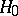: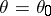against the alternative: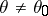. Suppose also that for a certain test with significance levelthere are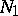observations needed to achieve a poweragainst the given alternativeand that another test of the same level needs for this purpose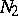observations. Then one can define the relative efficiency of the first test with respect to the second by the formula. The concept of relative efficiency gives exhaustive information for the comparison of tests, but proves to be inconvenient for applications, since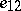is a function of the three arguments,andand, as a rule, does not lend itself to computation in explicit form. To overcome this difficulty one uses a passage to a limit.

The quantity, for fixedand(if the limit exists), is called the asymptotic relative efficiency in the sense of Pitman. Similarly one defines the asymptotic relative efficiency in the sense of Bahadur, where for fixed,the limit is taken astends to zero, and the asymptotic relative efficiency in the sense of Hodges and Lehmann, when for fixedandone computes the limit as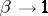.

Each of these definitions has its own merits and shortfalls. For example, the Pitman efficiency is, as a rule, easier to calculate than the Bahadur one (the calculation of the latter involves the non-trivial problem of studying the asymptotic probability of large deviations of test statistics); however, in a number of cases it turns out to be a less sensitive tool for the comparison of two tests.

Suppose, for example, that the observations are distributed according to the normal law with averageand variance 1 and that the hypothesis:is to be verified against the alternative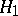: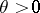. Suppose also that one considers a significance test based on a sample mean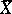and Student ratio. Since the-test does not use information on the variance, the optimal test must be that based on. However, from the point of view of Pitman efficiency these tests are equivalent. On the other hand, the Bahadur efficiency of the-test in relation tois strictly less than 1 for any.

In more complicated cases the Pitman efficiency may depend onorand its calculation becomes very tedious. Then one calculates its limiting value asor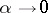. The latter usually is the same as the limiting value of the Bahadur efficiency as.

For other approaches to the definition of asymptotic efficiency of a test see ; sequential analogues of this concept are introduced in . The choice of one definition or another must be based on which of them gives a more accurate approximation to the relative efficiency; however, at present (1988) little is known in this direction .